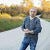# A Quick Lesson in Overhead

When Going Faster Slows You Down

Usually when building a machine learning algorithm from scratch (like we do in the Cornell Machine Learning program), you want to employ vectorized / matrixed math operations everywhere you possibly can in order to speed up performance. The reason for this is that it’s faster, in aggregate, to perform 100 calculations simultaneously than it is to perform the same type of calculation 100 times. Take for example, the image below, in which I take a 100-dimensional array of data and multiply it by a set of weights, and then do the same thing with 100 instances of 100-dimensional data against the same weights.

Even though the second operation did 100x more calculations, the total wall time was just about 2x the time it took to do a single operation.

This sometimes leads to the erroneous conclusion that a matrix math approach is *always* preferred, when possible. However, it turns out that this depends on the nature of the problem you’re trying to solve.

Take, for example, the Perceptron. The basic logic behind it in a binary classification challenge is to evaluate some number of points looking for misclassification, and when you find one, you adjust your weights and keep moving down the line. After some number of modifications you eventually reach the end of the list, and then you start over at the beginning and iterate through the entire list again performing the same steps. You do this until you finally get all the way through the entire list of data points without needing to make a weight update, at which point those weights become the source of the math behind your predictions.

Some students like to try to speed this up by using matrix multiplication / inner products. Why not try all data points at once, rather than iterate one at a time? Then, you can likely achieve convergence in a smaller number of iterations (which may be true, but is not guaranteed) and it will be faster (which may not be true, and as we will demonstrate, is a dubious assumption at best.)

The challenge to this approach is two-fold. One, you can only perform one weight update at a time, regardless of the number of misclassified points per iteration, because as soon as you’ve adjusted for a single point, the math changes for all other points in the matrix. Two — while you may be able to get to convergence in fewer iterations, it may still be slower than the one-at-a-time approach.

While it is true that you can do 100 calculations faster using matrix multiplication / inner products, it is not exactly the same speed as doing a single calculation. As we noted, it’s approximately double the time in the example I created. Now then, given the overhead involved in computing a result for 100 observations **at each iteration** it becomes a guessing game as to how many fewer iterations you have to perform and whether that ends up actually saving you any time or not.

To illustrate, I built a simple function and did some tests (which you can download or view here) in which I took an input of data, weights, and number of assumed iterations. It turned out that extending my example above, for my 100-dimensional data, the break-even point was about 30:

Thus, if the math operation being performed took 30 or more iterations, it would usually take more compute time than taking 100 iterations on a single data point at a time.

Different hardware would produce different results, and the more complex the operation, the wider the difference might be as well. In which direction, I couldn’t tell you. The main point here is to be careful about making assumptions. When you have a theory about algorithm performance, test it out, and specifically try to design a test that proves your preconcieved notion wrong. It’s going to teach you something in the process, regardless of where the true answer lies, so the exploration itself ande the related learning is really the end goal. The fact that it makes you a better programmer / data scientist / engineer in the process is just gravy.

--

--## Jason Eden

Data Science & Cloud nerd with a passion for making complex topics easier to understand. All writings and associated errors are my own doing, not work-related.